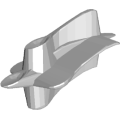CGAL 4.8.2 - 3D Minkowski Sum of Polyhedra
3D Minkowski Sum of Polyhedra ReferencePeter Hachenberger
This package provides a function, which computes the Minkowski sum of two point sets in $$\mathbb{R}^3$$. These point sets may consist of isolated vertices, isolated edges, surfaces with convex facets without holes, and open and closed solids. Thus, it is possible to compute the configuration space of translational robots (even in tight passage scenarios) as well as several graphics operations, like for instance the glide operation, which computes the point set swept by a polyhedron that moves along a polygonal line.

Introduced in: CGAL 3.5
Depends on: 3D Boolean Operations on Nef Polyhedra, Convex Decomposition of Polyhedra
BibTeX: cgal:h-msp3-16b
Windows Demo: Operations on Polyhedra
Common Demo Dlls: dlls

## Functions

• CGAL::minkowski_sum_3()

## Functions

template<typename Nef_polyhedron_3 >
Nef_polyhedron_3 CGAL::minkowski_sum_3 (Nef_polyhedron_3 &N0, Nef_polyhedron_3 &N1)
The function minkowski_sum_3() computes the Minkowski sum of two given 3D Nef polyhedra $$N0$$ and $$N1$$. More...

## Function Documentation

template<typename Nef_polyhedron_3 >
 Nef_polyhedron_3 CGAL::minkowski_sum_3 ( Nef_polyhedron_3 & N0, Nef_polyhedron_3 & N1 )

The function minkowski_sum_3() computes the Minkowski sum of two given 3D Nef polyhedra $$N0$$ and $$N1$$.

Note that the function runs in $$O(n^3m^3)$$ time in the worst case, where $$n$$ and $$m$$ are the complexities of the two input polyhedra (the complexity of a Nef_polyhedron_3 is the sum of its Vertices, Halfedges and SHalfedges).

An input polyhedron may consist of:

1. singular vertices
2. singular edges
3. singular convex facets without holes
4. surfaces with convex facets that have no holes.
5. three-dimensional features, whose coplanar facets have common selection marks (this includes open and closed solids)

Taking a different viewpoint, the implementation is restricted as follows:

1. The input polyhedra must be bounded (selected outer volume is ignored).
2. All sets of coplanar facets of a full-dimensional feature must have the same selection mark (in case of different selection marks, unselected is assumed).
3. All facets of lower-dimensional features need to be convex and must not have holes (non-convex facets and holes are ignored).
Postcondition
If either of the input polyhedra is non-convex, it is modified during the computation, i.e., it is decomposed into convex pieces.
CGAL::Nef_polyhedron_3<Traits>
CGAL::convex_decomposition_3()
#include <CGAL/minkowski_sum_3.h>